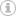# Math tricks 01 – multiplying a number by 11 in mind

When I was in middle school, I was taught some tricks to do multiplication in mind quickly. I don’t think here in US math teacher will teach (maybe they don’t know) kids about any tricks and I would like to share some of the tricks here; hopefully it will help someone.

How to multiply a number by 11 in mind quickly?

For example, 11 X 35. Here is the trick:

1. The first digit of 35 will be the first digit of the result

2. The last digit of 35 will be the last digit of the result

3. The middle digit of the result will be the sum of 3 and 5.

In other words, you stretch the number 35, then insert the sum of 3 and 5 between 3 and 5, and the result will 385, as shown in the following picture.If the summation in the step 3 above is equal to or greater than 10, than keep the ones digit of the sum, and add the tens digit (which is 1) to the digit in the step 1 above, as shown in the following picture.You can extend this trick to the multiplication of a three-digit number and 11. For example, 235 X 11 = 2585. The middle digits in the result are from 2+3 and 3+5 respectively. However, if any summation of the middle digits is equal to or greater than 10, then keep the ones digit and add the tens digit to the next summation. Keep it in mind that the summation needs to be done from the rightmost to the leftmost. For example, 3+5 goes before 2+3.

357 X 11 = 3927 ===> 3 (3+5) (5+7) 7 ===> 3(8) (12) 7 ===> 3 (9)(2)7===>3927

458 X 11 = 5038 ===> 4 (4+5) (5+8) 8 ===> 4 (9) (13) 8 ===>4 (10) (3) 8 ==>5 (0)(3)8 ===>5038

5 1 vote
Article Rating
Subscribe
Notify of1 Comment
Inline FeedbackswpDiscuz